# GED Math : Proportions and Percentages

## Example Questions

### Example Question #31 : Proportions And Percentages

Currently, Brian pays $865 a month for his rent. Monthly rent is due to increase by 12% starting next month. Rounded to the nearest dollar, how much will he pay then? Possible Answers: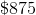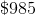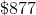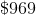Correct answer:Explanation: 12% of$865 is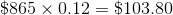.

Add that amount to the current rent: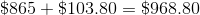This rounds to $969, the correct choice. ### Example Question #32 : Proportions And Percentages Thomas’s current yearly salary is$44,000 per year. After an 8% raise, what will his new salary be?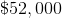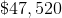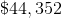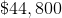Explanation:

8% of Thomas's salary of $44,000 is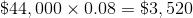. Add this to the original salary: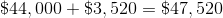### Example Question #33 : Proportions And Percentages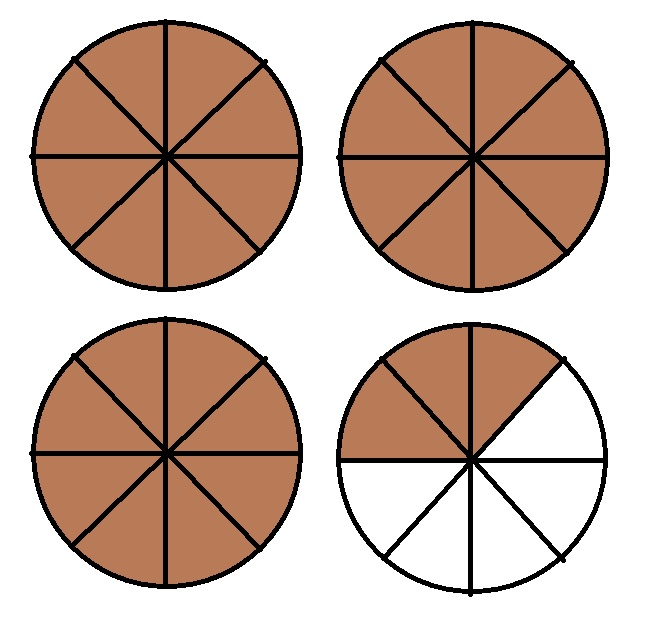Refer to the above diagram. The circles are of equal size and the portions of each circle are of equal size. If the unshaded portion of the above diagram were to be shaded, then, to the nearest tenth, by what percent would the area of the shaded region increase? Possible Answers: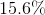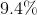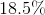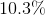Correct answer:Explanation: At current, 27 regions of equal size are shaded. If the remainder of the diagram were shaded, this would increase the size of the shaded portion by 5 regions of the same size. Therefore, we need to calculate what percent 5 is of 27. This is an increase in area of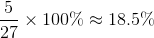. ### Example Question #34 : Proportions And Percentages Greg deposits$9,000 at 7% simple interest per year. How much will he have in this account after eighteen months, assuming he does not make any withdrawals or deposits?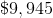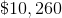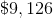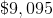Explanation:

Use the simple interest formula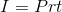with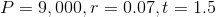(18 months = 1.5 years):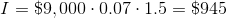This is the simple interest earned. Add it to the original principal: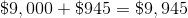### Example Question #35 : Proportions And Percentages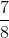of a circle is black; the rest is white. What percent of the circle is white?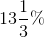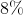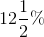Explanation:

Ifof a circle is black, then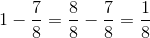of the circle is white., as a percent, is equal to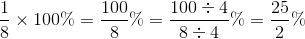.

Since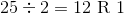,

this amount converts to.

### Example Question #31 : Proportions And Percentages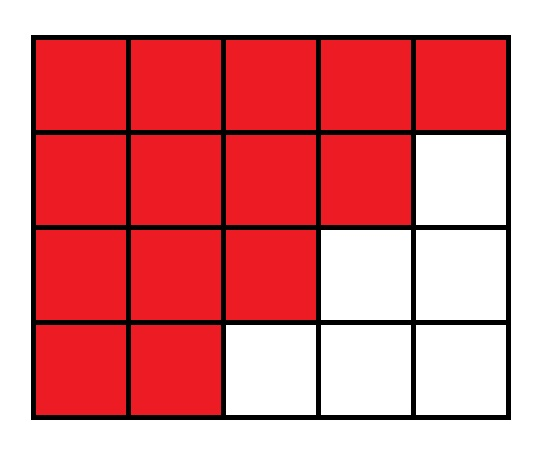Refer to the above diagram. The portions of the large rectangle are of equal size.

If the unshaded portions of the above diagram were to be shaded, then, to the nearest tenth, by what percent would the area of the shaded region increase?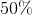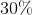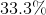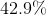Explanation:

At current, 14 regions of equal size are shaded. If the remainder of the squares were shaded, this would increase the size of the shaded portion by 6 regions of the same size. Therefore, we need to calculate what percent 6 is of 14. This is an increase in area of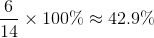.

### Example Question #401 : Ged Math

Gerald's current monthly rent is $720. His rent will increase by 20% starting in January; it will increase by$50 starting in July. How much rent will Gerald pay from January to December?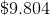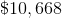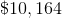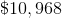Explanation:

If Gerald's current rent is $720, then a 20% increase in rent will be an increase of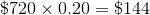. This makes Gerald's rent from January to June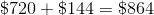. His rent from July to December will be$50 more than this, or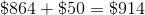.

Over the next year, Gerald will pay $864 in monthly rent for six months, then$914 in monthly rent for six months. This is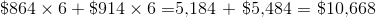in rent total.

### Example Question #401 : Ged Math

Quinn invests $6,000 in stocks and bonds. He invests 60% of the money in corporate bonds which, over the next year, return 8%. The remainder, he invests in a preferred stock, which ends up producing a loss of 4%. How much money has Quinn gained or lost from his investments? Possible Answers: Quinn has lost$384

Quinn has lost $192 Quinn has gained$384

Quinn has gained $192 Correct answer: Quinn has gained$192

Explanation:

60% of 6,000 is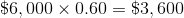, so this is what Quinn has invested in the bonds. The bonds return 8% of this, or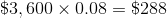.

Quinn invests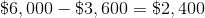in stock. It yields a loss of 4% of this, or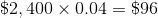.

Quinn has a net gain of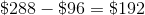from his investments.

### Example Question #403 : Ged Math

Seven tenths of three fourths ofis what percent of?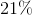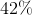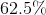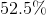Explanation:

Seven tenths of three fourths ofis equal to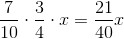.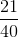of a number, converted to percent, is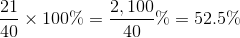.

### Example Question #71 : Complex Operations

What is 40% of 80% of?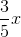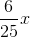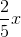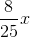80% ofis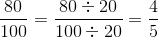multiplied by, or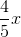.
40% of this is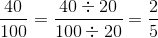multiplied by; this is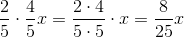.